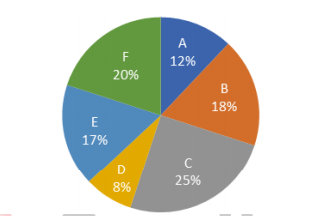## Questions On Quantitative Techniques SMQTQ024Directions (Q.1-5):- Study the following pie chart carefully and answer the questions given beside.

The following pie chart gives the information about breakup of six different categories of workers in a company1. In category A, the ratio of male workers to female workers is 2 : 3. The number of female workers in category A is what percentage of the total number of workers in category F?

A. 35.5%
B. 36%
C. 37.5%
D. 38%

2. The number of Category B workers is what percentage more than that of category D workers?

A. 125%
B. 120%
C. 100%
D. 140%

3. One day, all the workers were present except 25 out of 102 of category E workers. Find the number of workers present on that day?

A. 600
B. 625
C. 575
D. 550

4. If total 1800 workers are working in the company then find the absolute difference between the number of workers in category D and category C ?

A. 306
B. 323
C. 289
D. 286

5. Which of the following three categories workers together is 60% of the total number of workers working in that company?

A. A, B, and F
B. A, B, and C
C. B, C, and E
D. B, C, and F